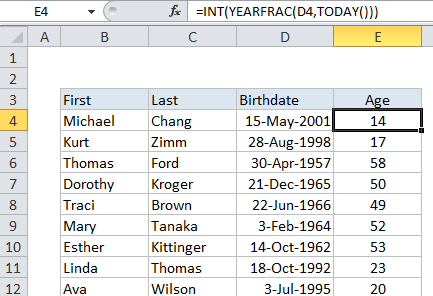## Excel Office

Excel How Tos, Tutorials, Tips & Tricks, Shortcuts

# Get date from day number in Excel

This tutorial show how to get date from day number in Excel using the example below.

To get a real date from day number, or “nth day of year” you can use the DATE function.

## Formula

`=DATE(year,1,daynum)`## Explanation of how this formula works

In the example shown, the formula in C5 is:

`=DATE(2015,1,B5)`

The DATE function build dates from separate year, month, and day values. One of it’s tricks is the ability to roll forward to correct dates when given days and months that are “out of range”.

Worked Example:   Add years to date in Excel

For example, DATE returns April 9, 2016 with the following arguments:

`=DATE(2016,1,100)`

There is no 100th day in January, so DATE simple moves forward 100 days from January 1 and figures returns the correct date.

Worked Example:   How to split text string at specific character in Excel

The formula on this page takes advantage of this behavior. The year assumed to be 2015 in this case, so 2015 is hard-coded for year, and 1 is used for month. The day value comes from column B, and the DATE function calculates the date as explained above.

### Incase you want to extracting a year value from a Julian date

If you have a date in a Julian format, for example, 10015, where the format is “dddyy”, you can adapt the formula as follows:

`=DATE(RIGHT(A1,2),1,LEFT(A1,3))`

Here, we use RIGHT to extract the 2 characters from the right for year, and LEFT to extract 3 characters from the left for day. Month is supplied as 1, like the first example.

Worked Example:   How to get top level domain (TLD) in Excel